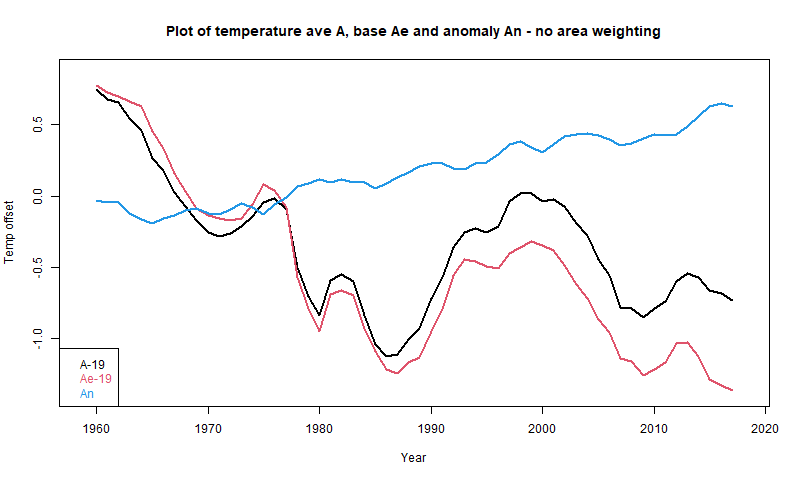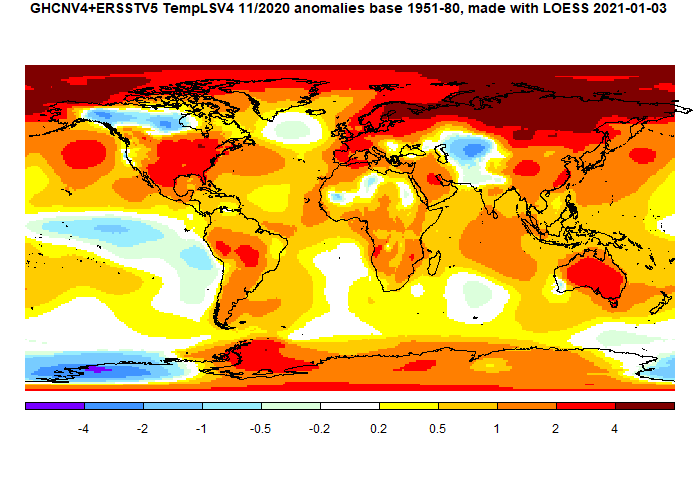Sunday, December 27, 2020

How averaging absolute temperatures goes wrong - use anomalies

There has been a series of posts at WUWT by Andy May on SST averaging, initially comparing HADSST with ERSST. They are here, here, here, here, and here. Naturally I have been involved in the discussions; so has John Kennedy. There has also been Twitter discussion.My initial comment was here
"Just another in an endless series of why you should never average absolute temperatures. They are too inhomogeneous, and you are at the mercy of however your sample worked out. Just don’t do it. Take anomalies first. They are much more homogeneous, and all the stuff about masks and missing grids won’t matter. That is what every sensible scientist does.
..."

The trend was toward HADSST and a claim that SST had been rather substantially declining this century (based on that flaky averaging of absolute temperatures). It was noted that ERSST does not show the same thing. The reason is that HADSST has missing data, while ERSST interpolates. The problem is mainly due to that interaction of missing cells with the inhomogeneity of T.

Here is one of Andy's graphs:In these circumstances I usually repeat the calculation that was done replacing the time varying data with some fixed average for each location to show that you get the same claimed pattern. It seems to me obvious that if unchanging data can produce that trend, then the trend is not due to any climate change (there is none) but to the range of locations included in each average, which is the only thing that varies. However at WUWT one meets an avalanche of irrelevancies - maybe the base period had some special property, or maybe it isn't well enough known, the data is manipulated etc etc. I think this is silly, because they key fact is that some set of unchanging temperatures did produce that pattern. So you certainly can't claim that it must have been due to climate change. I set out that in a comment here, with this graph:Here A is Andy's average, An is the anomaly average, and Ae is the average made from the fixed base (1961-90) values. Although no individual location in Ae is changing, it descends even faster than A.

So I tried another tack. Using base values is the simplest way to see it, but one can just do a partition of the original arithmetic, and along the way find a useful way of showing the components of the average that Andy is calculating. I set out a first rendition of that here. I'll expand on that here, with a more systematic notation and some tables. For simplicity, I will omit area weighting of cells, as Andy did for the early posts.

Breakdown of the anomaly difference between 2001 and 2018

Consider three subsets of the cell/month entries (cemos):
• Ra is the set with data in both 2001 and 2018 (Na cemos)
• Rb is the set with data in 2001 but not in 2018 (Nb cemos)
• Rc is the set with data in 2018 but not in 2001 (Nc cemos)
I'll mark sums S of cemo data with a 1 for 2001, 2 for 2018, and an a,b,c if they are sums for a subset. I use a similar notation for averages with A plus suffices. I'll set out the notation and some values in a table:

 Set data N Weights 2001 2018 2001 or 2018 N=18229 S1, A1=S1/(Na+Nb)=19.029 S2,A2=S2/(Na+Nc)=18.216 Ra 2001 and 2018 Na=15026 Wa=Na/N=0.824 S1a, A1a=S1a/Na=19.61 S2a, A2a=S2a/Na=19.863 Rb 2001 but not 2018 Nb=1023 Wb=Nb/N=0.056 S1b, A1b=S1b/Nb=10.52 S2b=0 Rc 2018 but not 2001 Nc=2010 Wc=Nc/N=0.120 S1c=0 S2b, A2b=S2b/Nb=6.865

I haven't given values for the sums S, but you can work them out from the A and N. The point is that they are additive, and this can be used to form Andy's A2-A1 as a weighted sum of the other averages. From additive S:
S1=S1a+S1b and S2=S2a+S2c
or
A1*(Na+Nb)=A1a*Na+A1b*Nb, or
A1*N=A1a*Na+A1b*Nb+A1*Nc
and similarly
A2*N=A2a*Na+A2c*Nc+A2*Nb
Differencing
(A2-A1)*N=(A2a-A1a)*Na-(A1b-A2)*Nb+(A2c-A1)*Nc
or, dividing by N

A2-A1=(A2a-A1a)*Wa-(A1b-A2)*Wb+(A2c-A1)*Wc

That expresses A2-A1 as the weighted sum of three terms relating to Ra, Rb and Rc respectively. Looking at these individually
• (A2a-A1a)=0.253 are the differences between the data points known for both years. They are the meaningful change measures, and give a positive result
• (A1b-A2)=-7.696. The 2001 readings in Rb have no counterpart in 2018, and so no information about increment. Instead they appear as the difference with the 2018 average A2. This isn't a climate change difference, but just reflects whether the points in R2 were from warm or cool places/seasons.
• (A2b-A1)=12.164. Likewise these Rc readings in 2018 have no balance in 2001, and just appear relative to overall A1.
Note that the second and third terms are not related to CC increases and are large, although this is ameliorated by their smallish weighting. The overall sum that, with weights, makes up the difference is
A2-A1 = 0.210 + 0.431 -1.455 = -0.813
So the first term representing actual changes is overwhelmed by the other two, which are biases caused by the changing cell population. This turns a small increase into a large decrease.

So why do anomalies help

I'll form anomalies by subtracting from each cemo the 2001-2018 mean for that cemo (chosen to ensure all N cemo's have data there). The resulting table has the same form, but very different numbers:

 Set data N Weights 2001 2018 2001 or 2018 N=18229 S1, A1=S1/(Na+Nb)=-.116 S2,A2=S2/(Na+Nc)=0.136 Ra 2001 and 2018 Na=15026 Wa=Na/N=0.824 S1a, A1a=S1a/Na=-0.118 S2a, A2a=S2a/Na=0.137 Rb 2001 but not 2018 Nb=1023 Wb=Nb/N=0.056 S1b, A1b=S1b/Nb=-0.084 S2b=0 Rc 2018 but not 2001 Nc=2010 Wc=Nc/N=0.120 S1c=0 S2b, A2b=S2b/Nb=0.130

The main thing to note is that the numbers are all much smaller. That is both because the range of anomalies is much smaller than absolute temperatures, but also, they are more homogeneous, and so more likely to cancel in a sum. The corresponding terms in the weighted sum making up A2-A1 are

A2-A1 = 0.210 + 0.012 + 0.029 = 0.251

The first term is exactly the same as without anomalies. Because it is the difference of T at the same cemo, subtracting the same base from each makes no change to the difference. And it is the term we want.

The second and third spurious terms are still spurious, but very much smaller. And this would be true for any reasonably choice of anomaly base.

So why not just restrict to Ra?

where both 2001 and 2018 have values? For a pairwise comparison, you can do this. But to draw a time series, that would restrict to cemos that have no missing values, which would be excessive. Anomalies avoid this with a small error.

However, you can do better with infilling. Naive anomalies, as used un HADCRUT 4 say, effectively assign to missing cells the average anomaly of the remainder. It is much better to infill with an estimate from local information. This was in effect the Cowtan and Way improvement to HADCRUT. The uses of infilling are described here (with links).

Tuesday, December 15, 2020

GISS November global up by 0.25°C from October.

The GISS V4 land/ocean temperature anomaly was 1.13°C in November 2020, up from 0.88°C in October. That compares with a 0.188deg;C rise in the TempLS V4 mesh index. It was the warmest November in the record.

Jim Hansen's update, with many more details, is here. He thinks that it is clear that 2020 will pass 2016 as hottest year.

As usual here, I will compare the GISS and earlier TempLS plots below the jump.

Saturday, December 5, 2020

November global surface TempLS up 0.188°C from October.

The TempLS mesh anomaly (1961-90 base) was 0.891deg;C in November vs 0.703°C in October. This rise was a little greater than the rise in the NCEP/NCAR reanalysis base index, which was 0.145°C. The UAH satellite data for the lower troposphere was little changed from October (but October was very warm). The Eastern Pacific ENSO region was cool.

It was the warmest month since November 2018, the second warmest November in the record (just behind 2015), and makes it likely that in this record, 2020 will be warmer than 2016, and hence the warmest full year in the record. The mean to November is 0.873°C, vs 2016 0.857, so December only has to be moderately warm for that to happen - in fact 0.681°C would be enough. I see the betting odds on that event are only 42% - or course they are not based on TempLS.

 Housekeeping note For the last six years I have made three global temperature postings every month - the NCEP results, then the TempLS results, and finally the comparison with GISS. But recently the GHCN data are posted so promptly that they are available almost as soon as NCEP. So I will in future merge the first two postings; no separate posting for NCEP.

There was a cool region in N Canada, but warm in the USA. Mainly, it was very warm in the Arctic, with an adjacent very warm region right across Eurasia. Most of the rest of the land was warm, including Antarctica. There was a cool area in central Asia.

Here is the temperature map, using the LOESS-based map of anomalies.As always, the 3D globe map gives better detail.